# Trigonometry Formulas and Identities, Check Concepts, Tips, and Practice Questions

Safalta expert Published by: Saksham Chauhan Updated Thu, 25 Aug 2022 02:04 AM IST

## Highlights

See the article below for the trigonometry formulae and trigonometry formula tricks. Candidates should review this information for tips on using trigonometric formulas.

One area of mathematics that involves a lot of formulae is trigonometry, and you can only solve questions on this subject if you are well-versed in the formulas and identities. There are various trigonometric ratios, formulas, and identities, including half-angle, double-angle, product, sum, and difference identities. It is crucial to comprehend and memorise these identities if you wish to learn trigonometry.Here we have provided the list of all important trigonometric formulas, identities, and more. If you are preparing for competitive exams and are looking for expert guidance, you can check out our monthly FREE Current Affairs E-book- Download Now. Also,  download our app- 'Exam Taiyari App' and boost your preparation. FREE GK EBook- Download Now.

Also check ,
Percentage Formula , Steps to calculate percentage
Geometry Definition, Formulas, and Shapes: What Is It?

## Trigonometry Formulas 2022

There are six trigonometric operations: Sin, Cos, Tan, Sec, Cosec, and Cot. Trigonometric ratios can be used to derive the current length and angle. All trigonometry formulae, formula tricks, and questions are built upon these six functions. Aspirants have access to information about trigonometry, including formulas, tips for using trigonometry formulas, and questions. There are questions about trigonometry in several competitive exams, including the SSC, Railway, and others.

### Free Demo Classes

This website has notes on formula trigonometry that are useful for exams. You can use it to help you master the basic trigonometric formulas. Below is a complete collection of all the trigonometric formulas for classes 10 and 11, as well as for classes 12 and 13.

## Important Identities of Trigonometric Ratios

To learn the basics of trigonometry, you must be aware of all significant trigonometric ratios and their identities.

## Ratios in Trigonometry

We will make advantage of a right-angled triangle to learn several trigonometric ratio functions. Assume that the angle A of the right-angled triangle ABC is 90 degrees.

### Trigonometric ratio relationships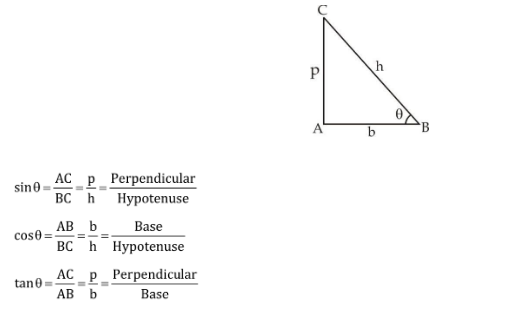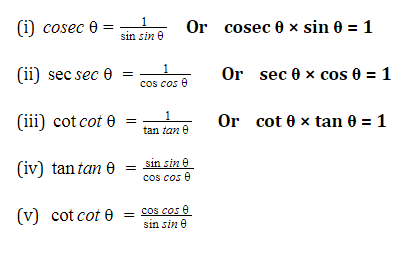## Trigonometric ratio values for each quadrant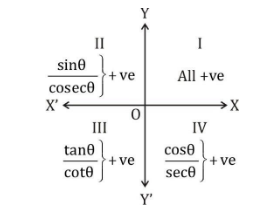## Different Trigonometric Ratio Specific Angle Values

To answer the question based on the trigonometric ratio of the angles 0°, 30°, 45°, and 60°, you must become familiar with the following table.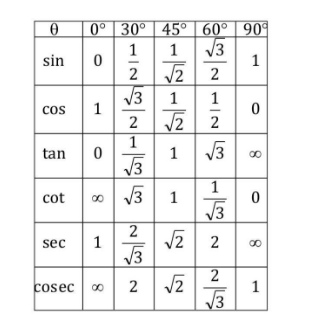## Relationship between the squares of several types of trigonometric ratios in trigonometry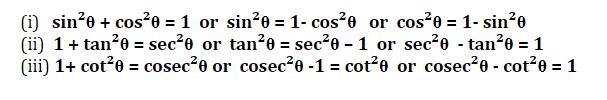## Important Idea to Address a Particular Type of Question

If A + B = 90°

Results that are true always :

(i)  sin A.

sec B = 1 or  sin A = cos B

(ii) cos A. cosec B = 1 or  sec A = cosec B

(iii) tan A. tan B = 1  or tan A = cot B

(iv) cot A. cot B = 1

(v)  sin²A + sin² B = 1

(vi) cos² A + cos² B = 1

Formula for the Sum and Difference of Two Angles in Trigonometry, an Important Formula

(1) sin (A+B) =sinA. cosB + cosA sinB

(2) sin(A – B) =sinA. cosB – cosA sinB

(3) cos(A+B) =cosA. cosB – sinA sinB

(4) cos(A-B) = cosA. cosB+sinA sinB

(5) 2 sinA.cosB = sin(A+B)+sin (A-B)

(6) 2 cosA. sinB = sin(A+B)-sin (A-B)

(7) 2 sinA. sinB = cos(A-B)-cos(A+B)

(8) 2 cosA.cosB = cos(A+B)+cos(A-B)

(9) sin²A-sin²B = sin(A+B). sin(A-B)

(10) cos²A-cos²B = cos(A+B).cos (A-B)

## Tangent Trigonometry Formulas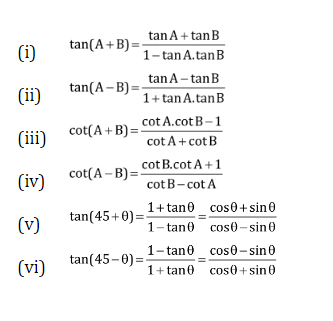List of trigonometric formulas

If A + B + C equals 180 degrees, then A + B + C equals A. B. C.

If A + B + C = 90°, then A + B + C must also equal A cot B cot C.

If sin(a) + cosec(a) = 2,

Then,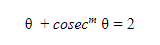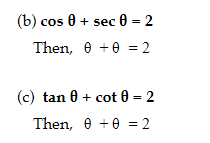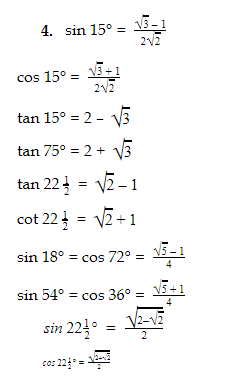## Maximum & Minimum Value in Trigonometry  Highest & Lowest Value

### The two extremes are Minimum and Maximum

Minimum                           Maximum

• sin θ, cos θ [odd power]             –1                                         +1
• sin θ, cos θ [even power]             0                                         +1
• tan θ, cot θ [odd power]             –∞                                       +∞
• tan θ, cot θ [even power]             0                                        +∞
• sec θ, cosec θ [odd power]         –∞                                       +∞
• sec θ, cosec θ [even power]        +1                                        +∞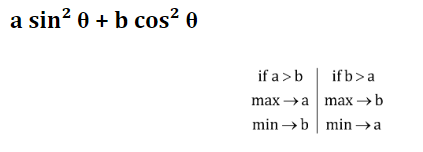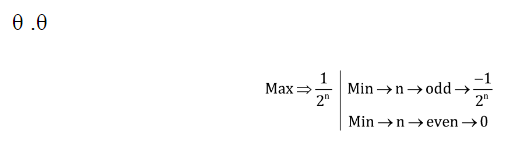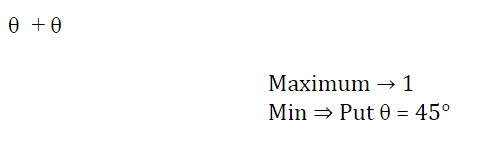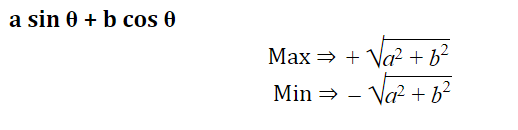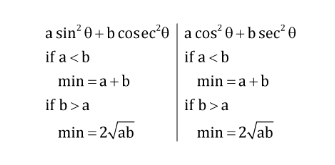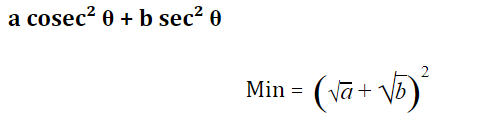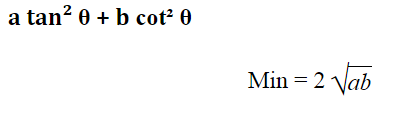## Who is the father of trigonometry?

Hipparchus of Nicaea

## What are the symbols in trigonometry?

Symbol Name Explanation Example
crd θ Chord function (Length of chord subtended by angle in unit circle) crd θ ≥ sin ⁡
Cosine function ⁡ θ + cos 2 ⁡
tan ⁡ Tangent function ⁡ ⁡ ⁡
Cosecant function ⁡ θ = 1 sin ⁡

Aryabhata I

## What is the Trigonometric ratio values for each quadrant ?